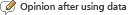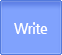연구성과물검색
유형별/분류별 연구성과물 검색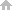HOME > Search by Achievements Type > Reports View

#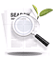Reports Detailed Information

자기상관이 있는 설명변수와 AR(p) 교란항이 있는 경우의 도구변수 추정에 관한 연구
Reports NRF is supported by Research Projects( 자기상관이 있는 설명변수와 AR(p) 교란항이 있는 경우의 도구변수 추정에 관한 연구 | 2006 Year | 김창진(고려대학교)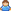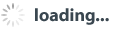) data is submitted to the NRF Project Results
Researcher who has been awarded a research grant by Humanities and Social Studies Support Program of NRF has to submit an end product within 6 months(* depend on the form of business)
• Researchers have entered the information directly to the NRF of Korea research support system
 Project Number B00275 Year(selected) 2006 Year the present condition of Project 종료 State of proposition 재단승인 Completion Date 2008년 04월 30일 Year type 결과보고 Year(final report) 2008년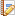Research Summary
•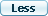Korean
• Reiersol (1941, 1945)과 와 Geary (1945) 에 의해서 도구변수 추정법 (instrumental variables estimation method)이 체계화 된 이후, 이단계 최소 자승법(two-stage least squares, 2SLS)은 계량 모형에서의 내생성을 해결하기 위해서 사용되어져 왔었다. 많은 시계열 모형에서도 설명변수와 잔차항 사이의 상관관계에서 비롯되는 내생성 문제가 종종 발생한다. 시계열 회귀분석에서 이런 내생성 문제가 존재할때, 설명변수에는 자기상관이 존재하지만 잔차항에 자기상관이 존재하지 않는다면, 설명변수들의 과거변수들로 이루어진 도구변수를 이용한 이단계 최소 자승법은 일치추정량(consistent estimator)을 제공한다. 그러나 만약 잔차항에 AR (autoregressive)이나 ARMA(autoregressive moving average) 유형으로 주어지는 자기상관이 존재한다면, 모형에 포함된 설명변수들의 과거치들은 유효한 도구변수가 될 수 없기 때문에 기존의 전통적인 이단계 최소자승법은 더이상 일치추정량을 제공할 수 없다.
따라서, 도구 변수 추정을 근거로 하는 기존의 모든 GMM (Generalized Method of Moments) 이나 이단계 추정법 (Two-Stage Least Squares, 2SLS)은 시계열 회귀식의 잔차항에 AR 이나 ARMA 유형의 자기상관이 존재하지 않는다고 가정하고 있다. 이러한 가정은 실제 현실과는 아주 다른 수 있다는 것은 아무도 부인하지 못할 것이다. 하지만 이러한 가정을 하지 않으면 설명변수나 종속변수의 과거치들을 도구변수로 사용할 수 없게되어 모형의 consistent한 추정 자체가 불가능하게 되기 때문에, 피할 수 없는 가정이었다. 본 연구에서는, 시계열 회귀 분석에서 설명변수와 종속변수들의 과거치 등을 도구변수를 사용하는 상황에서 잔차항에 AR 이나 ARMA 유형의 자기상관이 존재할 때, 모형을 consistent 하고 efficient 하게 추정할 수 있는 방법을 제시하고 있다. 문제의 해결은, 우선 잔차항에 자기상관이 없도록 모형을 변환한 후, control function approach를 도입하는 데 있다. 그러면 Heckman-type 이단계 추정이 가능해 진다. 본 논문에서 소개된 방법은 소표본에서도 큰 문제가 없다는 것을 몬테칼로 실험을 통하여 보여주고 있다.
•English
• In most time-series regression models with endogenous regressors, lagged regressors are commonly used as instrumental variables. Recent and important examples in empirical macroeconomics include estimation of the hybrid new Keynesian Phillips curve (Gali and Gertler (1999), new Keynesian IS curve (Fuhrer and Rudebusch (2004), and forward-looking monetary policy rule (Clarida, Gali, and Gertler (2000), etc. However, these instrumental variables are valid only under the assumption that the disturbance term in the regression equation of interest is serially uncorrelated. If the disturbance term is serially correlated with AR (augorgressive) or ARMA (autoregressive moving average) dynamics, conventional 2SLS (two stage least squares) or GMM (generalized method of moment) is not consistent because all the lagged regressors are invalid instruments.
To solve this problem, we propose a Heckman-type (1979) alternative two-step procedure. The idea is to decompose the disturbance term in the regression equation of interest into the two orthogonal portions: one that is correlated with the explanatory variables and the other that is not correlated with the explanatory variables. In the first step, we regress our endogenous explanatory variables on a set of instrumental variables including lagged regressors and get standardized residuals. In the second step, we incorporate the standardized residuals obtained from the first step as additional regressors in the regression equation to correct for the endogeneity problem and the serially correlated disturbances. These additional regressors, which are the portion of the disturbance term correlated with the existing regressors, act as bias correction terms and consistent estimates for the regression coefficients can be obtained. The second-step regression equation with these bias correction terms, modified to deal with serially correlated disturbances, can be estimated by either nonlinear least squares (NLS) or Quasi-maximum likelihood estimation (QMLE). Monte Carlo experiments confirm that the proposed two-step procedure performs well even for sample sizes as small as 250. A nice thing about the proposed methodology is its expandability. For example, under the normality assumption, it can easily be extended to deal with the endogeneity problem in the presence of heteroscedastic as well as autoregressive disturbance terms. The proposed two-step procedure would provide us with not only consistent but also efficient estimates of the coefficients of interest.Research result report
•Abstract
• We present a unified framework to solve the endogeneity problem in time-series regression models with serially correlated disturbances. Our focus is on the case in which lagged regressors are used as instrumental variables. While the conventional 2SLS (two-stage least squares) method fails to provide consistent estimates of the coefficients under this situation, we propose a two-step procedure based on Heckman’s (1979) original idea that provides efficient, as well as consistent, coefficient estimates. We also present a method for obtaining correct standard errors of the coefficients in the presence of ‘generated regressors’ in the second step regression, in light of Pagan (1984).
•Research result and Utilization method
• If there exist autoregressive disturbances in time series models with endogenous regressors, conventional 2SLS (two-stage least squares) or GMM (generalized methods of moments) using lagged regressors as instruments would result in inconsistent estimates. Recently, Kim, Osborn, and Zhang (2006) report statistically significant serial correlation in the disturbance terms of the stylized hybrid new Keynesian Phillips curves, casting doubt on the validity of these commonly used instrumental variables. Thus, an alternative two-step procedure introduced in this paper would shed new light on IV estimation of many important empirical macroeconomic time series models, in the presence of autoregressive disturbance terms.
Monte Carlo experiments confirm that the proposed two-step procedure performs well even for sample sizes as small as 250. A nice thing about the proposed methodology is its expandability. For example, under the normality assumption, it can easily be extended to deal with the endogeneity problem in the presence of heteroscedastic as well as autoregressive disturbance terms. The proposed two-step procedure would provide us with not only consistent but also efficient estimates of the coefficients of interest.
• Index terms
• Endogeneity, IV Estimation, Autoregressive Disturbance term, Alternative Two-Step Procedure, Generated Regressors
•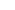List of digital content of this reports• This document, it is necessary to display the original author and you do not have permission